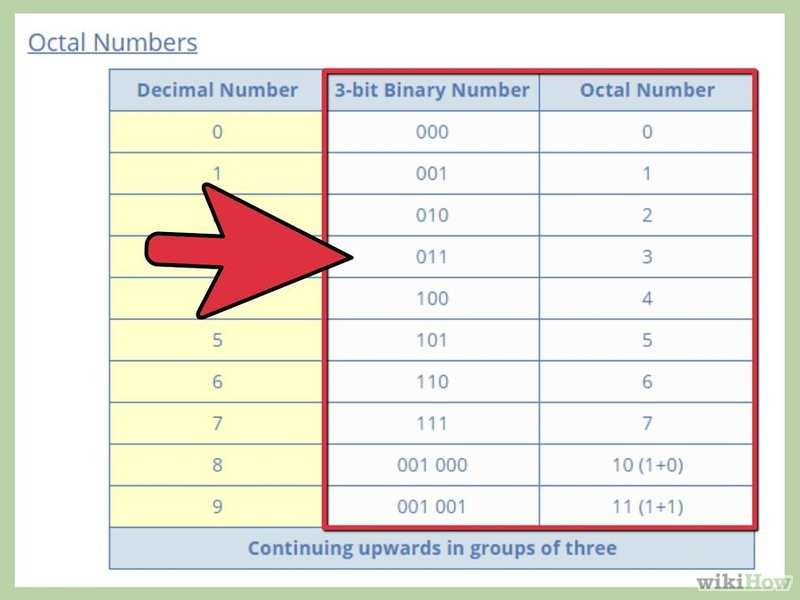Consider any binary number. A binary number consists of only 0's and 1's.

## Part 1

1

Group all the bits in the binary number, as a set of 3 bits.

Start from the right: approaching from least significant bit to the most significant bit.

• If any bit remains ungrouped in a set of 3 bits then you can add a leading '0' to it on the left, to make it a perfect set.

2

Find the octal numbers and their equivalent binary numbers, as given in the picture.

3

Replace each 3-bit binary number set to its equivalent octal number.

Hence the number obtained after replacing is the equivalent octal number.